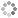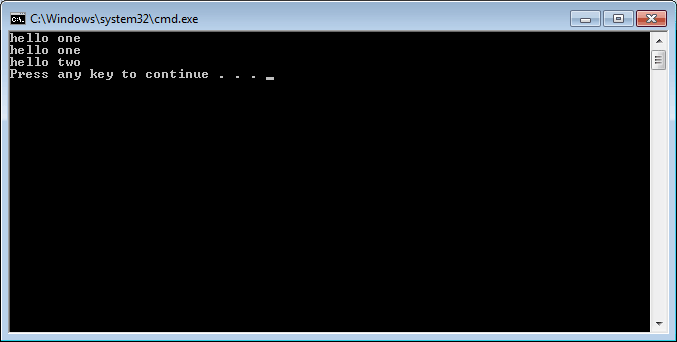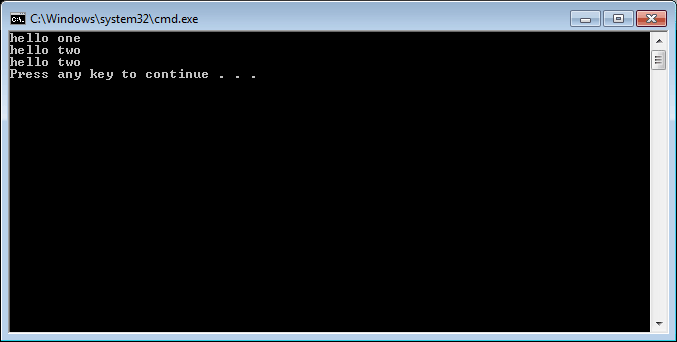# PROWAREtecharticles » current » c-plus-plus » polymorphism

## C++: Polymorphism

A quick example of how polymorphism works.

Polymorphism is a little over hyped. Code execution decisions are made at execution time instead of compilation time.

First an example that does not use polymorphism.

``````#include <iostream>
using namespace std;

class One
{
public:
void hello() { cout << "hello one" << endl; }
};

class Two : public One
{
public:
void hello() { cout << "hello two" << endl; }
};

int main()
{
One one;
one.hello();
One *pOne = new Two();
pOne->hello(); // executes One::hello(), determined at compile time
Two *pTwo = new Two();
pTwo->hello();

return 0;
}
``````Now, change the method in the base class to a virtual method and execute the code. This is late-binding/dynamic binding (decided at execution time). The method `hello()` is now polymorphic. This requires dynamic memory (memory not on the stack) using the `new` operator. The method `Two::hello()` has an "override" of `One::hello()`.

``````#include <iostream>
using namespace std;

class One
{
public:
virtual void hello() { cout << "hello one" << endl; }
};

class Two : public One
{
public:
void hello() { cout << "hello two" << endl; }
};

int main()
{
One one;
one.hello();
One *pOne = new Two();
pOne->hello(); // executes Two::hello(), determined at run time
Two *pTwo = new Two();
pTwo->hello();

return 0;
}
``````This site uses cookies. Cookies are simple text files stored on the user's computer. They are used for adding features and security to this site. Read the privacy policy.